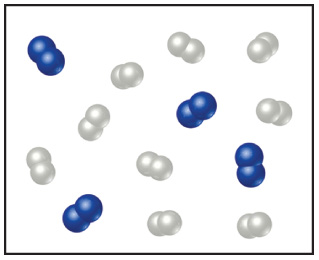# Problem: Nitrogen (N2) and hydrogen (H2) react to form ammonia (NH3). Consider the mixture of N2 and H2 shown in the accompanying diagram. The blue spheres represent N, and the white ones represent H.How many molecules of ammonia can be made, assuming the reaction goes to completion, based on the diagram?

###### FREE Expert Solution

We need to determine how many molecules of ammonia can be made from four molecules of nitrogen (N2) and nine molecules of hydrogen (H2).

We will solve this problem through these steps:

Step 1: Write a balanced equation for the formation of ammonia (NH3).

Step 2: Calculate the number of moles of NH3 from both reactants to determine the limiting reagent.

Step 3: Find out the number of moles of NH3 formed.

95% (171 ratings)###### Problem Details

Nitrogen (N2) and hydrogen (H2) react to form ammonia (NH3). Consider the mixture of N2 and H2 shown in the accompanying diagram. The blue spheres represent N, and the white ones represent H.How many molecules of ammonia can be made, assuming the reaction goes to completion, based on the diagram?